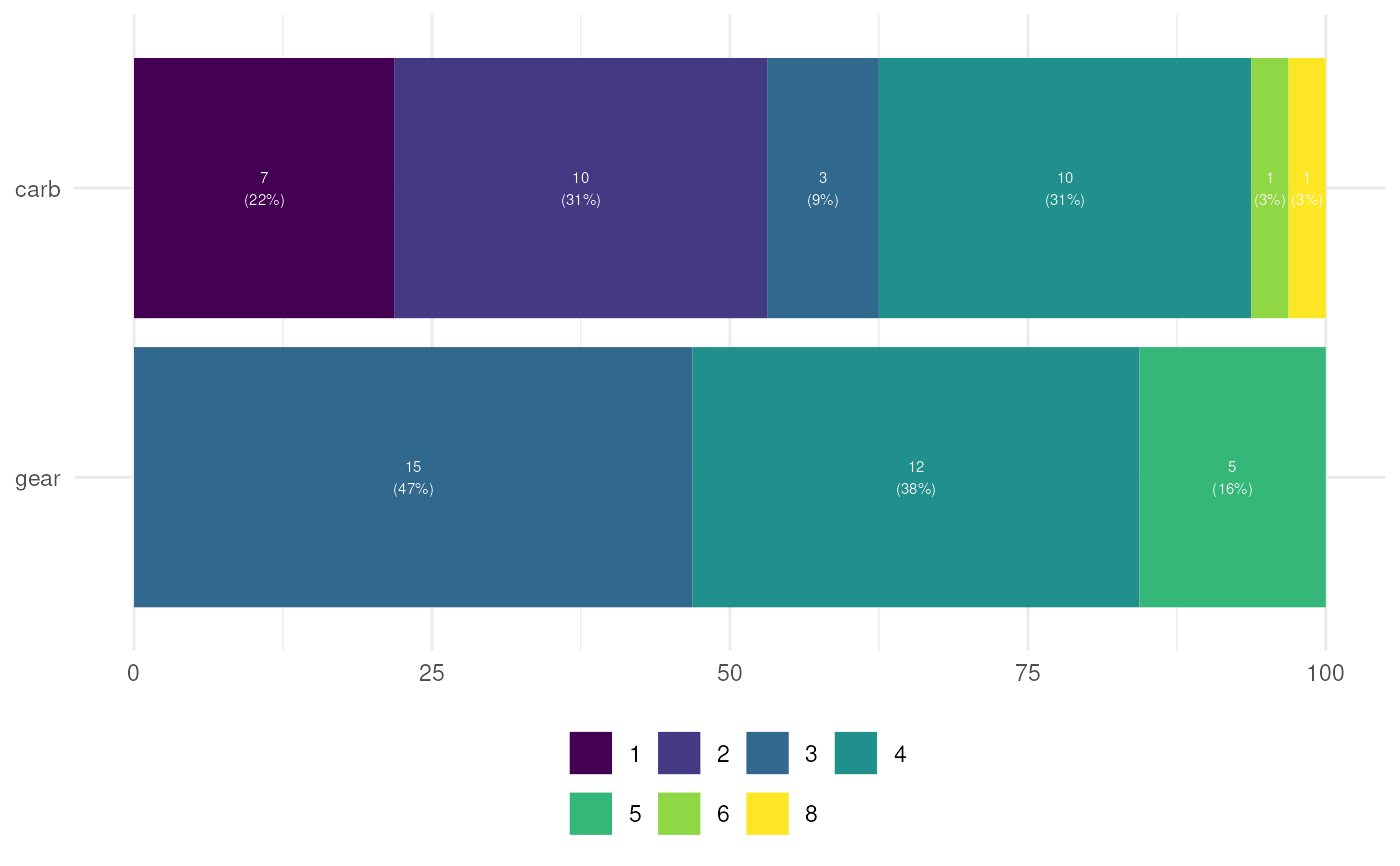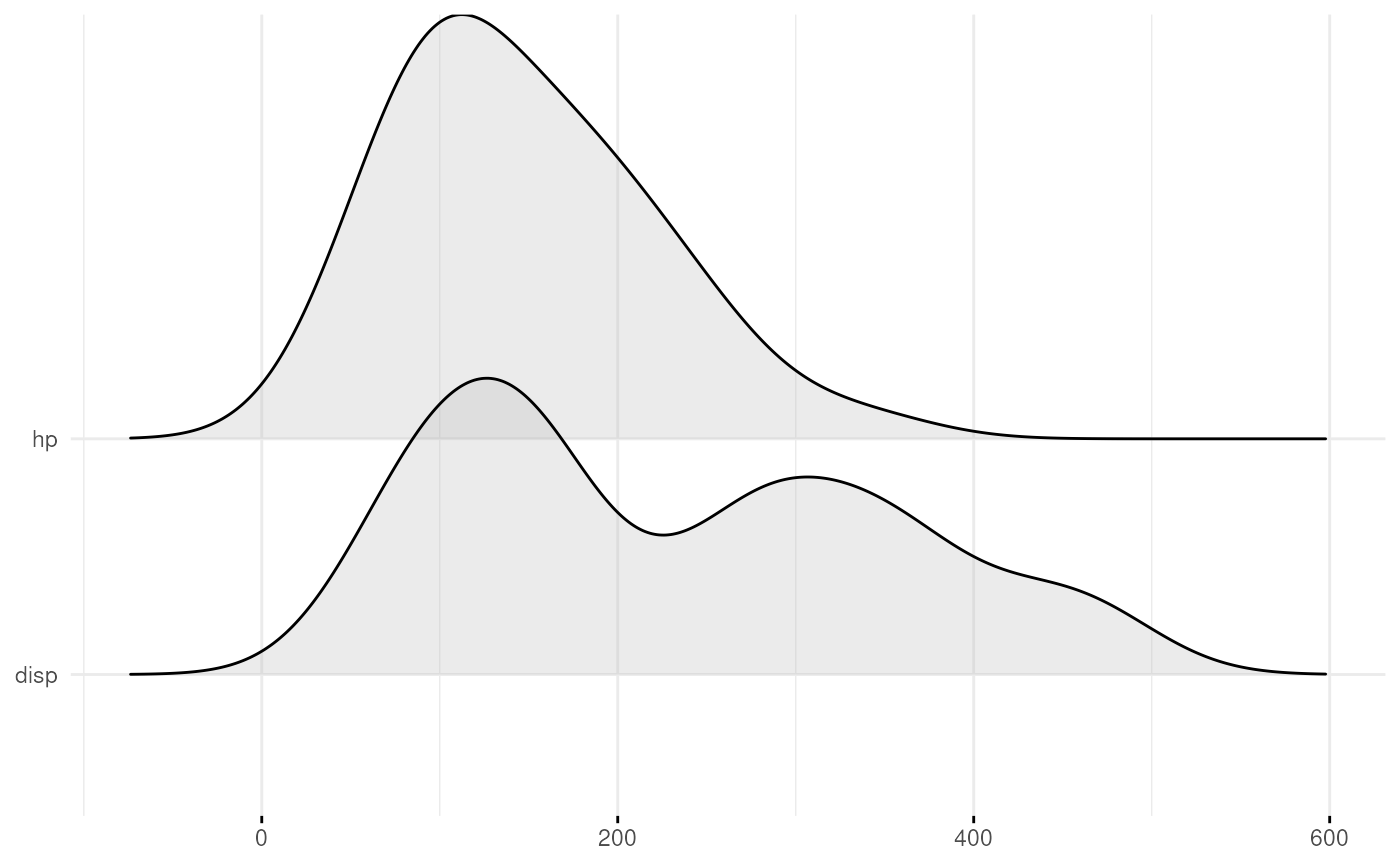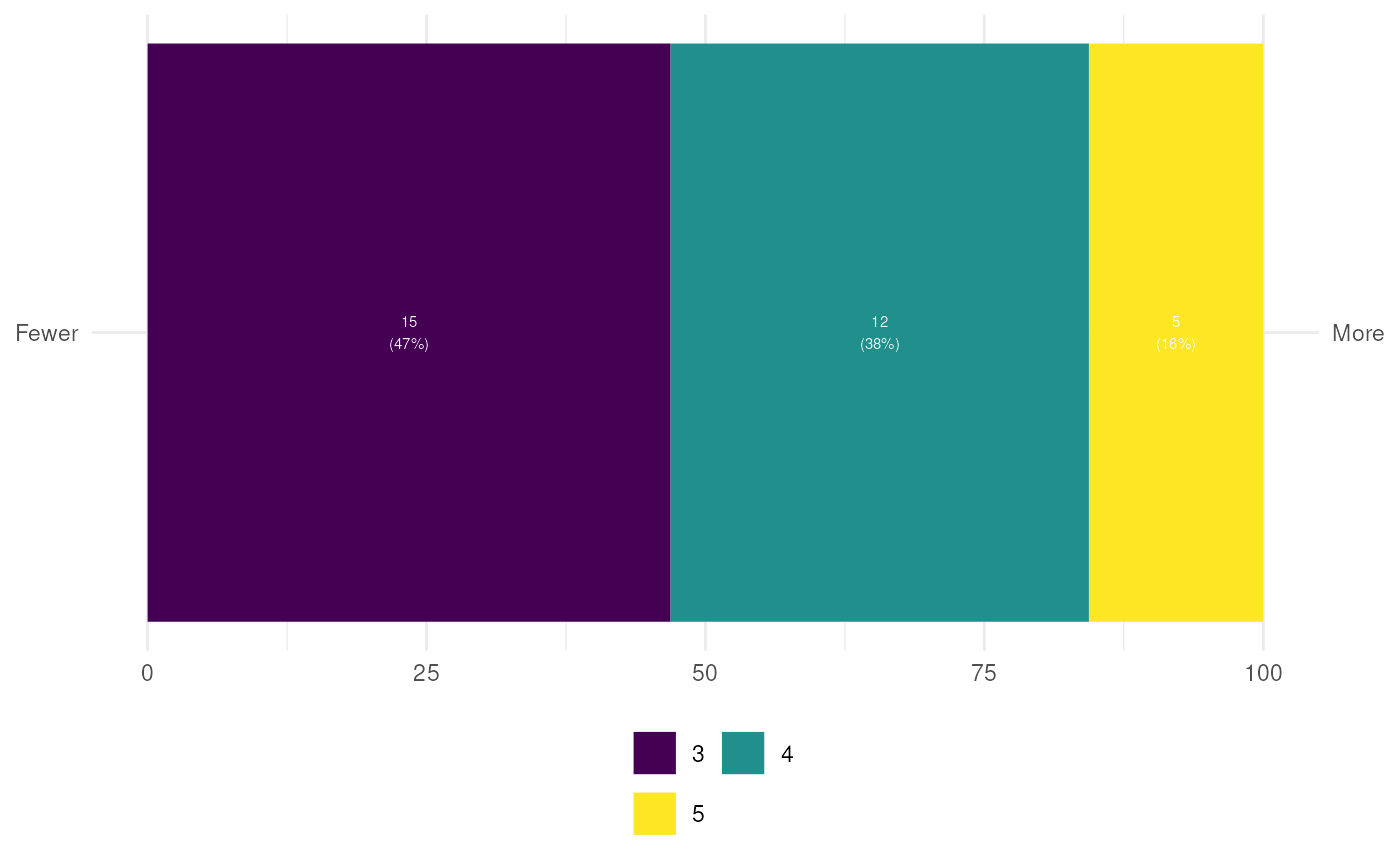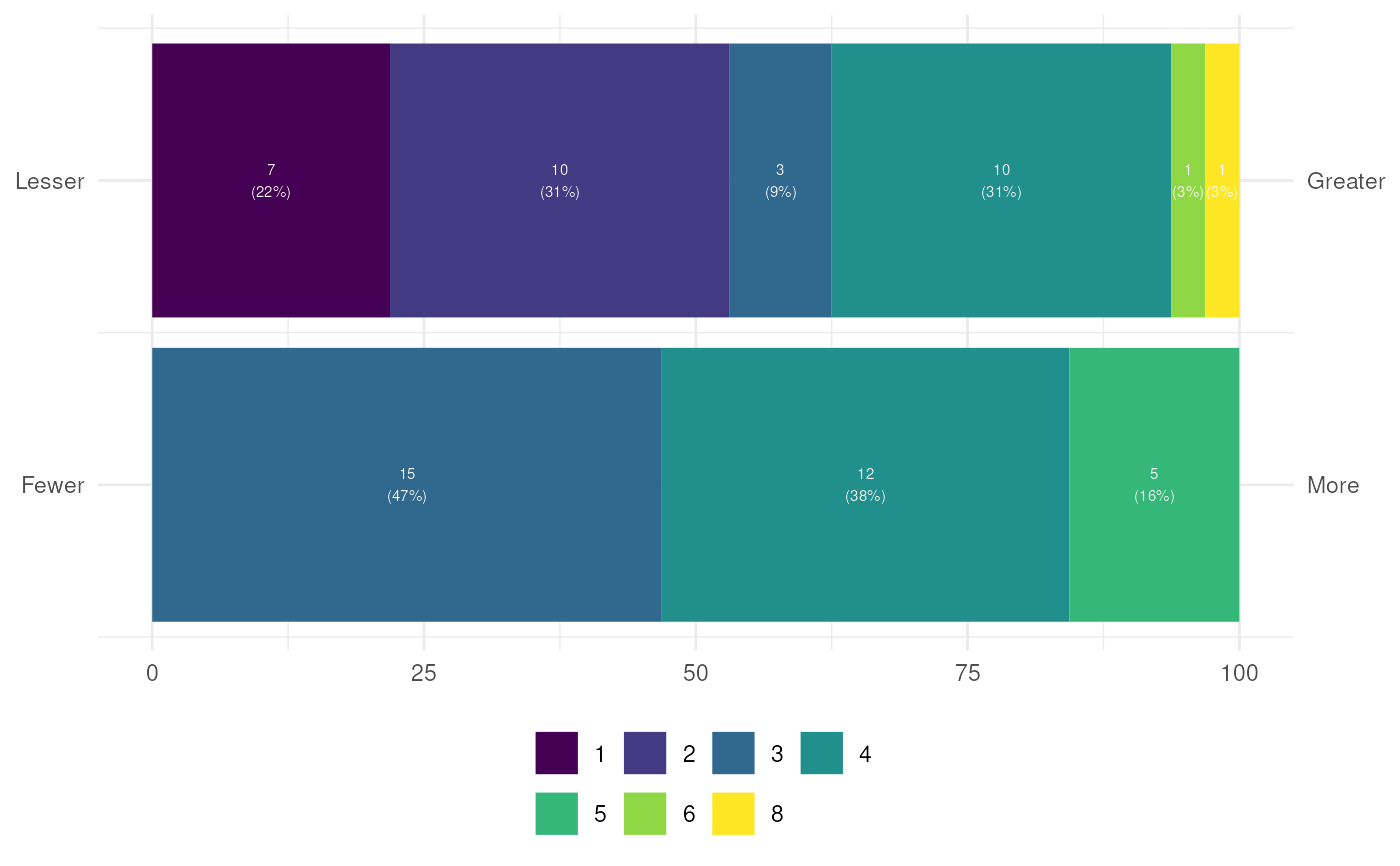These are convenience functions to quickly generate plots for multiple variables, with the variables in the y axis.

ggEasyBar(
data,
items = NULL,
labels = NULL,
sortByMean = TRUE,
xlab = NULL,
ylab = NULL,
scale_fill_function = NULL,
fontColor = "white",
fontSize = 2,
labelMinPercentage = 1,
showInLegend = "both",
legendRows = 2,
legendValueLabels = NULL,
biAxisLabels = NULL
)

ggEasyRidge(
data,
items = NULL,
labels = NULL,
sortByMean = TRUE,
xlab = NULL,
ylab = NULL
)

## Arguments

data

The dataframe containing the variables.

items

The variable names (if not provided, all variables will be used).

labels

Labels can optionally be provided; if they are, these will be used instead of the variable names.

sortByMean

Whether to sort the variables by mean value.

xlab, ylab

The labels for the x and y axes.

scale_fill_function

The function to pass to ggplot() to provide the colors of the bars. If NULL, set to ggplot2::scale_fill_viridis_d(labels = legendValueLabels, guide = ggplot2::guide_legend(title = NULL, nrow=legendRows, byrow=TRUE)).

fontColor, fontSize

The color and size of the font used to display the labels

labelMinPercentage

The minimum percentage that a category must reach before the label is printed (in whole percentages, i.e., on a scale from 0 to 100).

showInLegend

What to show in the legend in addition to the values; nothing ("none"), the frequencies ("freq"), the percentages ("perc"), or both ("both"). This is only used if only one variable is shown in the plot; afterwise, after all, the absolute frequencies and percentages differ for each variable.

legendRows

Number or rows in the legend.

legendValueLabels

Labels to use in the legend; must be a vector of the same length as the number of categories in the variables.

biAxisLabels

This can be used to specify labels to use if you want to use labels on both the left and right side. This is mostly useful when plotting single questions or semantic differentials. This must be a list with two character vectors, leftAnchors and rightAnchors, which must each have the same length as the number of items specified in items. See the examples for, well, examples.

## Value

A ggplot() plot is returned.

geom_ridgeline(), geom_bar()

## Author

Gjalt-Jorn Peters

Maintainer: Gjalt-Jorn Peters gjalt-jorn@userfriendlyscience.com

## Examples


ggEasyBar(mtcars, c('gear', 'carb'));ggEasyRidge(mtcars, c('disp', 'hp'));
#> Picking joint bandwidth of 41.9### When plotting single questions, if you want to show the anchors:
ggEasyBar(mtcars, c('gear'),
biAxisLabels=list(leftAnchors="Fewer",
rightAnchors="More"));### Or for multiple questions (for e.g. semantic differentials):
ggEasyBar(mtcars, c('gear', 'carb'),
biAxisLabels=list(leftAnchors=c("Fewer", "Lesser"),
rightAnchors=c("More", "Greater")));